﻿ Analytical Solutions of One-Dimensional Convection-Diffusion ProblemsPublications are Open
Access in this journal
Article Versions
Export Article
Cite this article
• Normal Style
• MLA Style
• APA Style
• Chicago Style
Research Article
Open Access Peer-reviewed

### Analytical Solutions of One-Dimensional Convection-Diffusion Problems

Hatıra Günerhan
Turkish Journal of Analysis and Number Theory. 2018, 6(6), 152-154. DOI: 10.12691/tjant-6-6-1
Received September 01, 2018; Revised October 04, 2018; Accepted November 05, 2018

### Abstract

This paper first describes RDTM and then uses Reduced RDTM to compute an approximate solution of the one-dimensional convection-diffusion problems. The method provides the solution quickly in the form of a convergent series. Our method reduces the computational difficulties existing in the other traditional methods. To show the efficacy of the results, three examples are considered.

### 1. Introduction

We consider the following one-dimensional convection-diffusion equation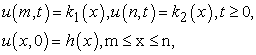(1.1)

Where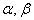andare known constant coefficients,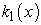and are known continuous functions.

The convection-diffusion equation is a merging of diffusion and convection equations, and describes the physical phenomena in which particles, energy, or other physical quantities are transmitted to the physical system due to the two processes of diffusion and convection.

In this study, firstly we describe the RDTM and then apply the RDTM to investigate the solution of the one-dimensional Convection-diffusion equation.

In recent years, some numerical solution have been developed to solve these types of convection-diffusion problems. Likes: the adaptive spline function approximation 1, several finite element methods 2, the finite difference approximation 3, cubic B-spline quasi-interpolation 4, restrictive taylor’s approximation 5, exponential B-spline collocation method 6. Exponential B-splines 7, weighted finite difference 8, meshless method 9, implicit method 10, redefined cubic B-splines collocation method 11. In 2009, the reduced differential transform method (RDTM) was introduced by Keskin 12 to solving solve various partial differential equations and fractional nonlinear partial differential equations. The technique of reduced differential transforms is a repetitive procedure for the solution of a Taylor series differential equations. This method reduces the size of the computational work and can be easily applied to many physical problems.

This paper has been organized as follows: Section 2 deals with the analysis of the method. In Section 3, we apply the RDTM to solve three types of one-dimensional Convection-Diffusion Problem. Conclusions are given in Section 4.

### 2. Reduced Differential Transform Method (RDTM)

Consider a function of two variablesand suppose that it can be represented as a product of two single-variable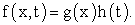the function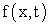can be represented as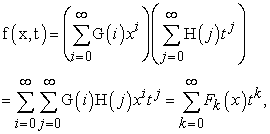(2.1)

Where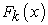is called t -dimensional spectrum function ofThe basic definitions and fundamental operations of the two-dimensional reduced differential transform method are introduced are defined in 13 as follows:

Definition 2.1. Assume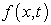is an analytic function in the domain of interest. The reduced differential transform ofis defined as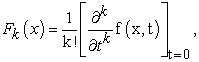(2.2)

Definition 2.2. The differential inverse transform ofis defined as follows: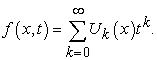(2.3)

By inserting equation (2.2) in (2.3), we obtain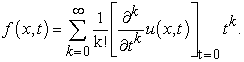(2.4)

Some basic properties of the two dimensional reduced differential transform are presented in Table 1 below.

### 3. Numerical Example

In this section, we used the basic definitions (in section 2) of the two-dimensional reduced differential transform method for solving three examples of the one-dimensional Convection-diffusion equations.

Example 1. We consider the following one-dimensional convection-diffusion problem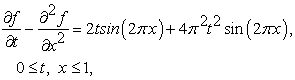(3.1)

with the boundary conditions and the initial condition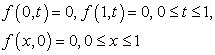(3.2)

by using the basic properties of RDTM in equations (3.1) and (3.2), we obtain the following relations(3.3)

From initial condition (3.2), we have(3.4)

Using Eq. (3.4) in Eq. (3.3), we get the following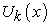values,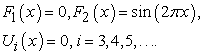(3.5)

By using the differential inverse reduced transform of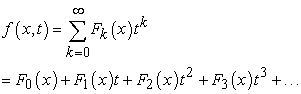(3.6)

we get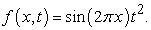(3.7)

We obtain the exact solution of equation (3.1).

Example 2. We consider the convection-diffusion(3.8)

and the boundary conditions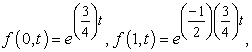(3.9)

subject to the initial conditions(3.10)

By using the basic properties of RDTM in equation (3.8), we obtain the following relations(3.11)

Taking the differential transform of Eqs.(3.10), we write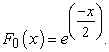(3.12)

Using Eq. (3.12) in Eq. (3.11), we get the followingvalues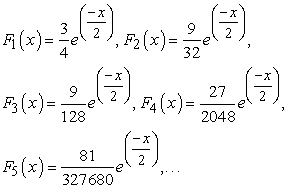(3.13)

the exact solution of the equation (3.8) will assume the following form:(3.14)

Example 3. Consider the convection-diffusion problem(3.15)

with the initial condition(3.16)

with the boundary condition:(3.17)

by using the basic properties of RDTM in equation (3.15) and (3.16), we obtain the following relations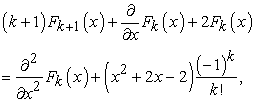(3.18)

and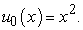(3.19)

Using Eq. (3.18) in Eq. (3.19), we get the followingvalues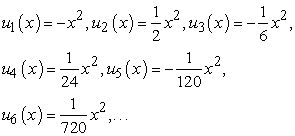(3.20)

By using the differential inverse reduced transform of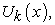we get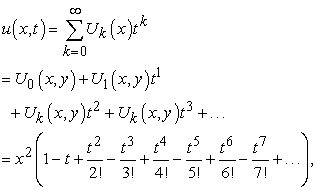(3.21)

by using the closed form in the solution of (3.21), we obtain the following exact solution(3.22)

### 4. Conclusion

In this work, we used reduced differential transform method (RDTM) to compute an approximate solution of the one-dimensional convection-diffusion problems. The method provides the solution in the form of a rapidly convergent series. Three explanatory examples are given to represent the efficacy of the obtained results.

### References

  Jain, M.K., Tariq, A., Numerical solution of stiff and convection-diffusion equations using adaptive spline function approximation, Applied Mathematical Modelling,7(1), 57-62, 1983. In article View Article  Codina, R., Comparison of Some Finite-Element Methods for Solving the Diffusion-Convection-Reaction Equation, Computer Methods in Applied Mechani – gineering, 156(1), 185-210,1998. In article  Salkuyeh, D.K.. On the finite difference approximation to the convection diffusion equation, Appl. Math. Comput, 179, 79-86, 2006. In article View Article  Aminikhah, H., and Alavi, J., Numerical Solution of Convection-Diffusion Equation Using Cubic B-Spline Quasi-Interpolation, Thai Journal of Mathematics, 14 (3), 599-613, 2016. In article  Ismail, H.N.A., Elbarbary, E.M.E., and Salem, G.S.E., Restrictive Taylor’s approximation for solving convection-diffusion equation, Appl. Math. Comput., 147, 355-363, 2004. In article View Article  Dehghan, M., On the Numerical solution of Dimensional Convection-Diffusion Equation, Mahtical Problems in Engineering, 2005(1), 61-74, 2005. In article View Article  Mohammadi, R., Exponential B-Spline Solution of Convection-Diffusion Equations, Applied Mathematics, 4, 933-944, 2013. In article View Article  Dehgan, M., Weighted Finite Difference Techniques for the One-Dimensional Advection Diffusion Equation, Applied Mathematics and Computation, 147(2), 307-319, 2004. In article View Article  LD, S., ZW, J., TS, J., Numerical Method for One-Dimensional Convection-diffusion Equation Using Radical Basis Functions, J Phys Math, 6(1), 136, 2015. In article  Ravi Kanth, A.S.V., a and Deepika, Numerical Solutions for Convection-Diffusion Equation through Non-Polynomial Spline, MATEC Web of Conferences 57, 05004, 2016. In article  Mittal, R.C. and Jain, R.K., Redefined Cubic B-Splines Collocation Method for Solving Convection-D usion Equations, Applied Mathematical Modelling, 36(11), 5555-5573, 2012, In article View Article  Keskin, Y., Ph.D Thesis, Selcuk University, Turkey, 2010. In article  Benhammouda, B., Vazquez-Leal, H., and Sarmiento-Reyes A., Modified Reduced Differential Transform Method for Partialm Differential-Algebraic Equations, SpringerPlus, 4(1), 1-9, 2015. In article

Published with license by Science and Education Publishing, Copyright © 2018 Hatıra GünerhanThis work is licensed under a Creative Commons Attribution 4.0 International License. To view a copy of this license, visit http://creativecommons.org/licenses/by/4.0/

### Cite this article:

##### Normal Style
Hatıra Günerhan. Analytical Solutions of One-Dimensional Convection-Diffusion Problems. Turkish Journal of Analysis and Number Theory. Vol. 6, No. 6, 2018, pp 152-154. http://pubs.sciepub.com/tjant/6/6/1
##### MLA Style
Günerhan, Hatıra. "Analytical Solutions of One-Dimensional Convection-Diffusion Problems." Turkish Journal of Analysis and Number Theory 6.6 (2018): 152-154.
##### APA Style
Günerhan, H. (2018). Analytical Solutions of One-Dimensional Convection-Diffusion Problems. Turkish Journal of Analysis and Number Theory, 6(6), 152-154.
##### Chicago Style
Günerhan, Hatıra. "Analytical Solutions of One-Dimensional Convection-Diffusion Problems." Turkish Journal of Analysis and Number Theory 6, no. 6 (2018): 152-154.
Share